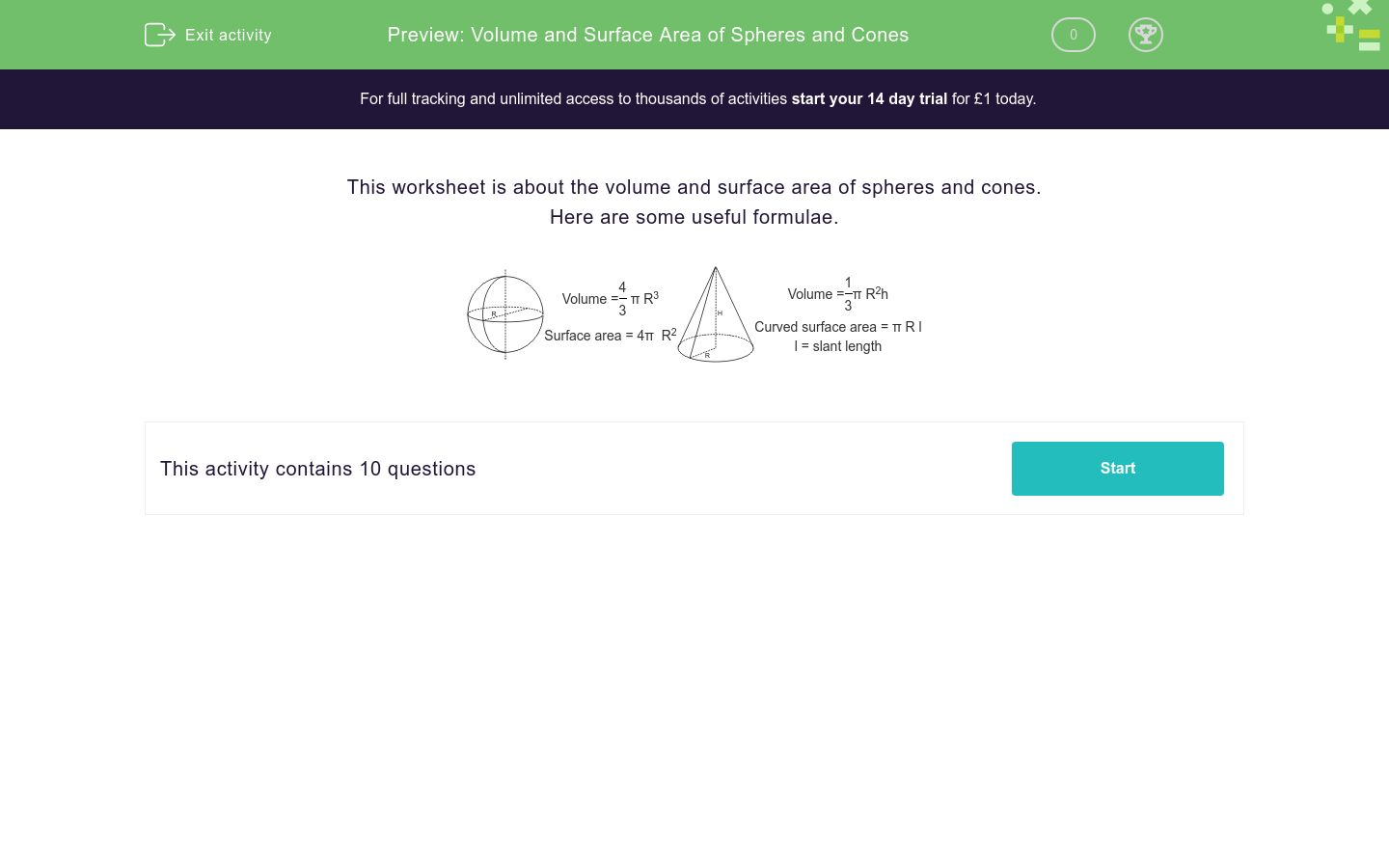# Volume and Surface Area of Spheres and Cones

In this worksheet, students find the volume and surface area of spheres and cones.Key stage:  KS 4

Curriculum topic:  Geometry and Measures

Difficulty level:### QUESTION 1 of 10

This worksheet is about the volume and surface area of spheres and cones.

Here are some useful formulae.Volume = 4 π R3 3
 Surface area = 4π  R2Volume = 1 π R2h 3
 Curved surface area = π R l l = slant lengthCalculate the volume of a sphere with radius 5 cm.

(Write your answer to 1 dp and just write the number)Calculate the surface area of a sphere with radius 5 cm.

(Write your answer to 1 dp and just write the number)1 2

The radius of Sphere 1 is 5 cm.

Calculate the surface area of a sphere with radius 3 times as big as that of Sphere 1.

(Write your answer to 1 dp and just write the number)1 2

The radius of Sphere 1 is 5 cm.

Calculate the volume of a sphere with radius 3 times as big as that of Sphere 1.

(Write your answer to 1 dp and just write the number)

Calculate the volume of a sphere with radius 10 cm.

(Write your answer to 1 dp and just write the number)

The radius of a sphere is 7 cm.

Calculate its volume.

(Write your answer to 1 dp and just write the number)Calculate the volume of a cone with radius 2 cm and height 6 cm.

(Write your answer to 1 dp and just write the number)Calculate the curved surface area of the cone with radius 3 cm and height 4 cm.

Hint: Use Pythagoras to calculate the slant length of the cone.

(Write your answer to 1 dp and just write the number)Calculate the volume of a cone with radius 3 cm and height 7 cm.

(Write your answer to 1 dp and just write the number)Calculate the curved surface area of the cone with radius 3 cm and height 7 cm.

Hint: Use Pythagoras to calculate the slant length of the cone.

(Write your answer to 1 dp and just write the number)

• Question 1Calculate the volume of a sphere with radius 5 cm.

(Write your answer to 1 dp and just write the number)

523.6
EDDIE SAYS
Vol = 4 ÷ 3 × π × 5³
• Question 2Calculate the surface area of a sphere with radius 5 cm.

(Write your answer to 1 dp and just write the number)

314.2
EDDIE SAYS
Surface area = 4 × π × 5²
• Question 31 2

The radius of Sphere 1 is 5 cm.

Calculate the surface area of a sphere with radius 3 times as big as that of Sphere 1.

(Write your answer to 1 dp and just write the number)

2827.4
EDDIE SAYS
Surface area = 4 × π × 15²
• Question 41 2

The radius of Sphere 1 is 5 cm.

Calculate the volume of a sphere with radius 3 times as big as that of Sphere 1.

(Write your answer to 1 dp and just write the number)

14137.2
EDDIE SAYS
Vol = 4 ÷ 3 × π × 15³
• Question 5

Calculate the volume of a sphere with radius 10 cm.

(Write your answer to 1 dp and just write the number)

4188.8
EDDIE SAYS
Vol = 4 ÷ 3 × π × 10³
• Question 6

The radius of a sphere is 7 cm.

Calculate its volume.

(Write your answer to 1 dp and just write the number)

1436.8
EDDIE SAYS
Vol = 4 ÷ 3 × π × 7³
• Question 7Calculate the volume of a cone with radius 2 cm and height 6 cm.

(Write your answer to 1 dp and just write the number)

25.1
EDDIE SAYS
Vol = 1 ÷ 3 × π × 2² × 6
• Question 8Calculate the curved surface area of the cone with radius 3 cm and height 4 cm.

Hint: Use Pythagoras to calculate the slant length of the cone.

(Write your answer to 1 dp and just write the number)

47.1
EDDIE SAYS
This is a 3, 4, 5 Pythagorean Triad.
Curved Surface Area = π × 3 × 5
• Question 9Calculate the volume of a cone with radius 3 cm and height 7 cm.

(Write your answer to 1 dp and just write the number)

66.0
EDDIE SAYS
Vol = 1 ÷ 3 × π × 3² × 7
• Question 10Calculate the curved surface area of the cone with radius 3 cm and height 7 cm.

Hint: Use Pythagoras to calculate the slant length of the cone.

(Write your answer to 1 dp and just write the number)